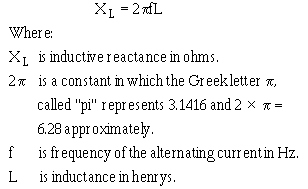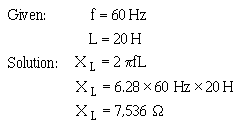inductance (L), the greater the opposition from this inertia effect. Also, the faster the reversal of current, the greater this inertial opposition. This opposing force which an inductor presents to the FLOW of alternating current cannot be called resistance, since it is not the result of friction within a conductor.">Custom SearchINDUCTIVE REACTANCE When the current flowing through an inductor continuously reverses itself, as in the case of an ac source, the inertia effect of the cemf is greater than with dc. The greater the amount of inductance (L), the greater the opposition from this inertia effect. Also, the faster the reversal of current, the greater this inertial opposition. This opposing force which an inductor presents to the FLOW of alternating current cannot be called resistance, since it is not the result of friction within a conductor. The name given to it is INDUCTIVE REACTANCE because it is the "reaction" of the inductor to the changing value of alternating current. Inductive reactance is measured in ohms and its symbol is XL. As you know, the induced voltage in a conductor is proportional to the rate at which magnetic lines of force cut the conductor. The greater the rate (the higher the frequency), the greater the cemf. Also, the induced voltage increases with an increase in inductance; the more ampere-turns, the greater the cemf. Reactance, then, increases with an increase of frequency and with an increase of inductance. The formula for inductive reactance is as follows:The following example problem illustrates the computation of X L.Q.3 What is the term for the opposition an inductor presents to ac? Q.4 What is the formula used to compute the value of this opposition? Q.5 What happens to the value of XL as frequency increases? Q.6 What happens to the value of XL as inductance decreases?Integrated Publishing, Inc. - A (SDVOSB) Service Disabled Veteran Owned Small Business